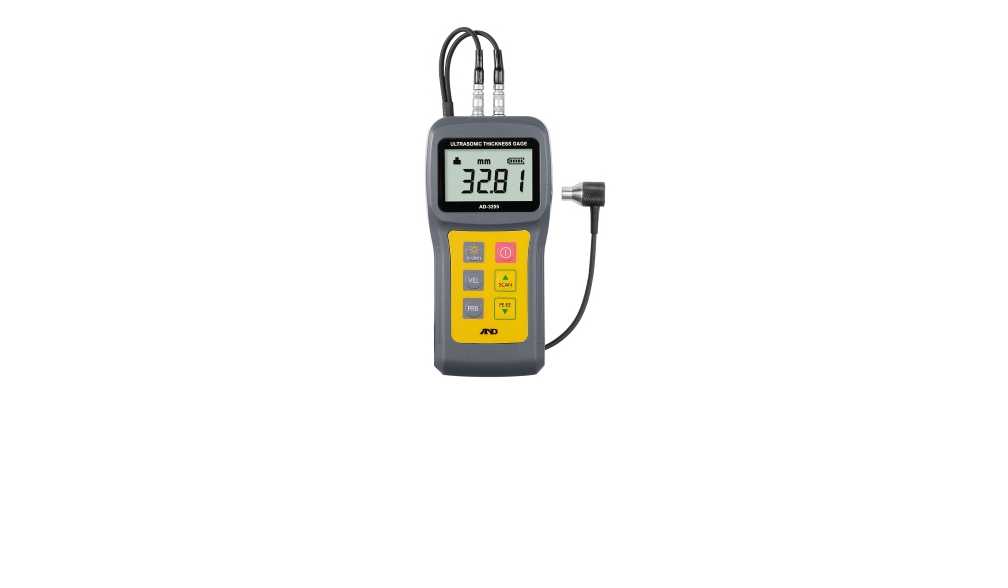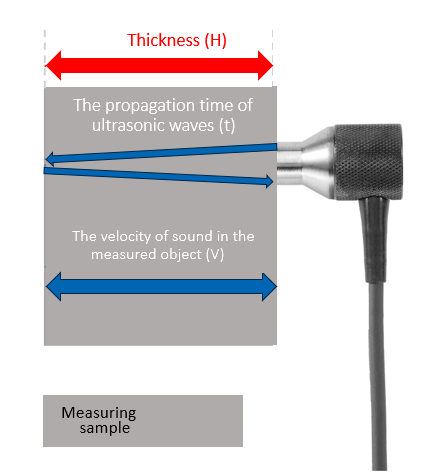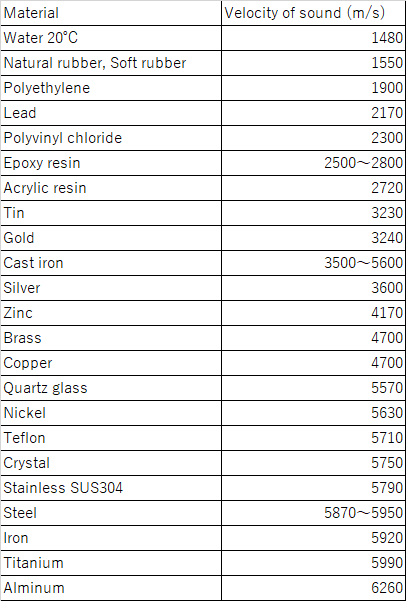By Akash Mendiratta

on Sat Sep 23 2023In an ultrasonic thickness gauge, ultrasonic waves emitted from a sensor called a probe are reflected

on the opposite side of the object to be measured.

Measure the propagation time until it returns to the probe again and calculate the thickness

The equation H (thickness) = v (velocity of sound) × t (propagation time) × 1/2 (refer to the diagram below)The velocity of sound varies depending on the type of material.
In ultrasonic thickness measurement, the thickness is calculated by multiplying the propagation time of ultrasonic waves

by the velocity of sound in the measured object.
To achieve accurate thickness measurement, it is crucial to have an accurate understanding of the correct velocity of sound.The diagram above is a list of the velocities of sound for various primary materials.

It is possible to research the velocity of sound for different materials through literature and online sources.

However, it is important to note that the velocity of sound can vary among different materials and even within the same material,

such as steel, where there can be a range of velocities.

Therefore, caution is necessary when considering the velocity of sound for specific materials.

If the velocity of sound in the measured object is unknown,

it is necessary to prepare a sample of the same material type with a known thickness.

In order to adjust the velocity of sound, the measurement needs to be conducted

under the same temperature conditions as the actual measurement.

There are two methods for adjusting the velocity of sound: single-point calibration and two-point calibration.

In single-point calibration, the zero-point calibration is performed first, followed by the measurement of the sample’s thickness.

By setting the value on the ultrasonic thickness gauge to match the actual thickness of the sample,

the velocity of sound in the sample is calculated.

In two-point calibration, additional measurements are taken at different thicknesses,

and the same procedure of setting the values is followed.

For AD-3255, it is possible to adjust the velocity of sound through single-point calibration,

two-point calibration, or manual numerical input.

Moreover, the settings and calibration data are stored in memory for future reference.